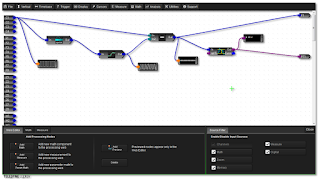## You need to test, we're here to help.

You need to test, we're here to help.

## 02 February 2018

### Getting The Most Out Of Your Oscilloscope: Math FunctionsFigure 1: Parameter math functionsprovide a way to create customparameters
Parameter math functions are an important part of an oscilloscope's analysis capabilities. Using parameter math, you can create custom parameters based on simple arithmetic relationships between existing parameters. It allows you to add, subtract, multiply, divide, or rescale parameters (Figure 1).

You can do things like inverting waveforms, multiply voltage and current to find power, or integrate a waveform to find energy and Joules. You can cascade measurements, or combine functions in a Math channel. Teledyne LeCroy's four-channel oscilloscopes provide eight Math channels; eight-channel instruments provide 12 Math channels.

With parameter math, you can combine parameters to calculate other parameters that Teledyne LeCroy oscilloscopes do not list in the parameter measurement listing, such as the crest factor of a waveform, or measuring the modulation index of an FM signal.Figure 2: WebEdit lets you create a graphical processing flowby dropping in math, measure, and parameter math operationsand connecting them together as necessary
If you need even more complex custom math functions, there's always the Processing Web Editor (or WebEdit for short). Let's face it: Waveform analysis is a complex affair in which you might need to link together a whole chain of math functions, parameters, and parameter math. WebEdit gives you a means of graphically constructing and modeling such math and measurement processing chains.

With WebEdit, you can cascade functions, or include things like Matlab scripts. There's almost no end to the possible combinations you can concoct with WebEdit. A preview function allows you to add little windows that will show you the output at the output of any operation, so you can check to see if what you're putting together is giving you what you expect it to, and this is independent of the oscilloscope application itself.Figure 3: Spectrum analysis software provides a user interfacefamiliar to users of traditional spectrum analyzers
The ability to convert a time-domain waveform acquisition to the frequency domain is an important tool for oscilloscope users. Fast Fourier transforms (FFTs) are not new to oscilloscopes, and Teledyne LeCroy offers FFT as a standard math operation on most of its oscilloscopes. But that capability goes a step further with its Spectrum Analysis option. The Spectrum Analysis software greatly simplifies the process of setting up an FFT by providing a user interface that is familiar to anyone who's spent time with traditional spectrum analyzers (Figure 3).

Setup of an FFT is pretty simple: Select a center frequency, span, and resolution bandwidth, and the software will set the proper sampling rate and time-domain acquisition length. An automatic peak-detect function identifies all frequency peaks, labels them on the display, and creates an interactive table of the peaks. You can also see the FFT as a spectrogram that shows how the spectra change over time.

We covered custom parameter measurements in an earlier post, but be aware that you also can set up user-defined math functions by selecting the Custom category in the Math-operator selection dialog. As with the custom parameters, custom Math operators supports Matlab, C/C++, Visual Basic scripts, VBA, Excel, and JavaScript. Custom Math lets you define your own scripts for waveform analysis and display of the results. It's fully integrated into the oscilloscope's processing architecture, so you can perform further analysis with parameters and so on.

We'll look at tools for the physical layer of serial protocols next.

Previous posts in this series:

Getting The Most Out Of Your Oscilloscope: Setup